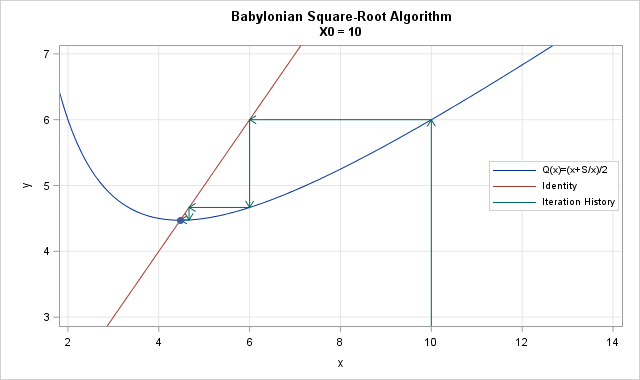I was eleven years old when I first saw Newton's method.

No, I didn't go to a school for geniuses. I didn't even know it was Newton's method until decades later. However, in sixth grade I learned an iterative algorithm that taught me (almost) everything I need to know about Newton's method for finding the roots of functions.

### Newton's method and an iterative square root algorithm

The algorithm I learned in the sixth grade is an iterative procedure for computing square roots by hand. It seems like magic because it estimates a square root from an arbitrary guess. Given a positive number S, you can guess any positive number x0 and then apply the "magic formula" xn+1 = (xn + S / xn)/2 until the iterates converge to the square root of S. For details, see my article about the Babylonian algorithm.

It turns out that this Babylonian square-root algorithm is a special case of Newton's general method for finding the roots of functions. To see this, define the function f(x) = x2 - S. Notice that the square root of S is the positive root of f. Newton's method says that you can find roots by forming the function Q(x) = x - f(x) / f′(x), where f′ is the derivative of f, which is 2x. With a little bit of algebra, you can show that Q(x) = (x + S / x)/2, which is exactly the "magic formula" in the Babylonian algorithm.

Therefore, the square root algorithm is exactly Newton's method applied to a special quadratic polynomial whose root is the square root of S. The Newton iteration process is visualized by the following graph (click to enlarge), which shows the iteration history of the initial guess 10 when S = 20.### Everything I need to know about Newton's method...

It turns out that almost everything I need to know about Newton's method, I learned in sixth grade. The Babylonian method for square roots provides practical tips about how to use Newton's method for finding roots:

• You need to make an initial guess.
• If you make a good initial guess, the iteration process is shorter.
• When you get close to a solution, the number of correct digits doubles for each iteration. This is quadratic convergence in action!
• Avoid inputs where the function is not defined. For the Babylonian method, you can't plug in x=0. In Newton's method, you need to avoid inputs where the derivative is close to zero.
• Different initial guesses might iterate to different roots. In the square root algorithm, a negative initial guess converges to the negative square root.

In fact, I'll go further. The Babylonian method teaches important lessons in numerical analysis:

• Root-finding is a fundamental tool that helps you solve all kinds of numerical problems.
• Many problems that do not have exact answers can be solved with iterative methods.
• Iterative methods often linearize the problem and use the linear formulation as part of the algorithm.

So you see, all I really needed to know about Newton's method I learned in sixth grade!

ShareDistinguished Researcher in Computational Statistics

Rick Wicklin, PhD, is a distinguished researcher in computational statistics at SAS and is a principal developer of SAS/IML software. His areas of expertise include computational statistics, simulation, statistical graphics, and modern methods in statistical data analysis. Rick is author of the books Statistical Programming with SAS/IML Software and Simulating Data with SAS.

1.•